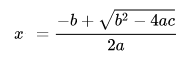# Class 11 Computer Science Sample Paper Term 2 Set-2

Subject Code: 083

Class: 11

Max Marks – 35 /Time – 02 Hrs.

General Instructions:

• The question paper is divided into 3 sections – A, B and C
• Section A, consists of 7 questions (1-7). Each question carries 2 marks.
• Section B, consists of 3 questions (8-10). Each question carries 3 marks.
• Section C, consists of 3 questions (11-13). Each question carries 4 marks.
•  Internal choices have been given for question numbers – 7, 8 and 12.

Section -A

Each question carries 2 marks

1. Write any two differences between lists and tuples.

Ans:

 List Tuple A list is mutable in nature A tuple is immutable in nature It is capable of consuming more memory It is capable of consuming less memory List iteration is more time consuming. Slower than tuple List iteration is less time consuming. Faster than a list

2. if L = [1,2,3,4,5,6,7,8], What will be the output of following statement?

i) L[: :-2]

ii) L[3: :2]

Ans:

i) [8, 6, 4, 2]

ii) [4, 6, 8]

3. What is a Dictionary? Can we define a list as a key of a dictionary?

Ans:

Dictionary is Mapping Mutable Data Types used to store the various value in pair (key:values) e.g.

d={1:”abc”, 2:”xyz”}

List cannot be used as key of dictionary because key is immutable.

4.Write equivalent python code of following expression :Ans:

x = (-b + math.sqrt(b * b – 4 * a * c)) / (2 * a)

5. Write the module /library import for following functions/methods:

i) mean( )

ii ) fabs( )

Ans:

i) statistics

ii) math

6. (i) What is the length of t1? t1= ((((1,2,3,4), “a”, “b”),”g”, 1, 3), “Sonu”)

Ans: 2

ii) Find and write the output of the following python code:
a=(“Amit”, “Brinda”,”Ashish”,”Sumanta”)
print(a.index(“Brinda”))

Ans: 1

7. Find and write the output of the following python code:
a=(1, 2, 3, 2, 3, 4, 5)
print(min(a) + max(a) + a.count(2))

Ans: 8

or

t1 = (23, 45, 67, 43, 21, 41)
print(t1[-1:-5:-1])

Ans: (41, 21, 43, 67)

Section -B

Each question carries 3 marks

8. Find and write the output of the following python code:
L =[“X”,20,”Y”,10,”Z”,30]
CNT = 0
ST = “”
INC = 0
for C in range(1,6,2):
CNT= CNT + C
ST= ST + L[C-1]+”@”
INC = INC + L[C]
print (CNT,INC,ST)

Ans:

1 20 X@

4 30 X@Y@

9 60 X@Y@Z@

or

Moves=[11, 22, 33]
Queen=Moves
Moves+=22
L=Len(Moves)
for i in range (L):
print “Now@”, Queen[L-i-1], “#”, Moves [i]

Ans:

Now@ 44 # 11

Now@ 55 # 22

Now@ 22 # 55

9. Write a Python Program to Input a tuple of elements, search for maximum element in the tuple and index of maximum element.

10. Find and write outputs of the following code:
Dc1={ }
Dc1=1
Dc1[“1”]=2
Dc1[1.0]=4
Sum=0
for k in Dc1:
Sum+=Dc1[k]
print(Sum)

Ans:

6

Section -C

Each question carries 4 marks

11. Write the python code to swap the list numbers in given manner:
e.g. [10,20,30,40,50]
Result: [50,40,30,20,10]

Ans:

L= [10,20,30,40,50]

l=[]

for i in range(len(L)):

l.append(L.pop())

L=l

print(L)

12. Find and write outputs of the following code. Also write the maximum and minimum value of pick.
import random
pick=random.randint(0,3)
city=[“Delhi”,”Mumbai”,”Chennai”,”Mumbai”]
for i in city:
for j in range(1,pick):
print(i,end=””)
print()

Ans:

DelhiDelhi

MumbaiMumbai

ChennaiChennai

MumbaiMumbai

and

Delhi

Mumbai

Chennai

Mumbai

or

Find and write outputs of the following code. Also write the maximum and minimum value of the picker.
import random
picker=1+random.randint(0,2)
color=[“Blue”,”Pink”,”Green”,”Red”]
for i in range(1,picker+1):
for j in range(i+1):
print(color[j],end=””)
print()

Ans:

BluePink

and

BluePink

BluePinkGreen

BluePinkGreenRed

13. Write a Python Program to Create a dictionary with the roll number, name and marks of n students in a class and display the names of students who have marks above 75.